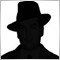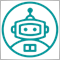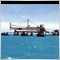• 概要
• レビュー (4)
• コメント
• 最新情報

# Extended Bollinger Bands MT5

This is an extended version of Bollinger Bands. You can use 21 variations of the moving average and 11 price options for the calculation of the middle line of the indicator. In addition, the period for calculating the standard deviation is defined by a separate parameter.

Moving Average types: Simple Moving Average, Exponential Moving Average, Wilder Exponential Moving Average, Linear Weighted Moving Average, Sine Weighted Moving Average, Triangular Moving Average, Least Square Moving Average (or EPMA, Linear Regression Line), Smoothed Moving Average, Hull Moving Average by Alan Hull, Zero-Lag Exponential Moving Average, Double Exponential Moving Average by Patrick Mulloy, T3 by T.Tillson, Instantaneous Trendline by J.Ehlers, Moving Median, Geometric Mean, Regularized EMA by Chris Satchwell, Integral of Linear Regression Slope, Combination of LSMA and ILRS, Triangular Moving Average generalized by J.Ehlers, Volume Weighted Moving Average, Smoothing by Mark Jurik.

Price options: Close, Open, High, Low, Median Price = (High+Low)/2, Typical Price = (High+Low+Close)/3, Weighted Close = (High+Low+Close*2)/4, Heiken Ashi Close, Heiken Ashi Open, Heiken Ashi High, Heiken Ashi Low.

### Parameters:

• PeriodMA - middle line calculation period,
• MethodMA - middle line smoothing method,
• PriceMA - price used for calculation,
• PeriodDev - period for calculation of the standard deviation,
• Deviations - the standard deviation multiplier,
• MethodDev - averaging method
• PriceDev - price for calculation of the standard deviation,
• ShiftMA - forward/backward shift of the indicator on the chart,
おすすめの製品
フィルタ:[削除された] 2019.01.14 06:27

ユーザーはレーティングに対して何もコメントを残しませんでした12010
2017.12.15 18:20

ユーザーはレーティングに対して何もコメントを残しませんでした5716
2017.11.09 13:42

ユーザーはレーティングに対して何もコメントを残しませんでした6208
2016.12.21 07:25

ユーザーはレーティングに対して何もコメントを残しませんでした

バージョン 1.5 2020.07.22
The code was optimized for the current version of the terminal.
バージョン 1.4 2016.02.09
Optimized the averaging calculation algorithm.
Added 5 new types of smoothing.
バージョン 1.3 2014.12.16